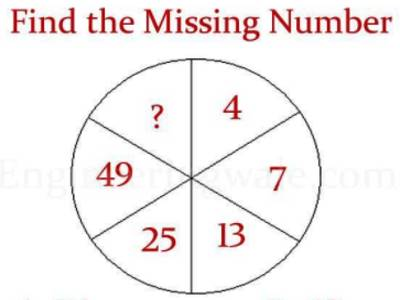Finding Missing Number in a Sequence

A Sequence is a set of things (usually numbers) that are in order. To find a missing number, first, find a Rule behind the Sequence.

How to find a missing number in a sequence

• Determine if the order of numbers is ascending (getting larger in value) or descending (becoming smaller in value).
• Find the difference between numbers that are next to each other.
• Use the difference between numbers to find the missing number.

Example: Find the missing number: 15, 13, ?, 9

• The order of numbers is going down or descending.
• The difference between numbers is 15 – 13 = 2
• Since the order is descending subtract 2 from 13. The missing number may be 11.
• The missing number is 11 since it is 2 more than the last number 9.

Example: Find the missing number: 30, 23, ?, 9

• The order of numbers is going down or descending.
• The difference between numbers is 30 – 23 = 7
• Since the order is descending subtract 7 from 23. The missing number may be 16.
• The missing number is 16 since it is 7 more than the last number 9.Sometimes we can just look at the numbers and see a pattern:

Example: 1, 4, 9, 16,… ?

Answer: they are Squares (12 =1, 22=4, 32=9, 42=16, …)

Rule: xn = n2

Sequence: 1, 4, 9, 16, 25, 36, 49, …

Example: 3, 5, 8, 13, 21, ?

After 3 and 5 all the rest are the sum of the two numbers before,

That is 3 + 5 = 8, 5 + 8 = 13 etc, which is part of the Fibonacci Sequence:

3, 5, 8, 13, 21, 34, 55, 89, …

Which has this Rule:

Rule: xn = xn-1 + xn-2

Information Source: